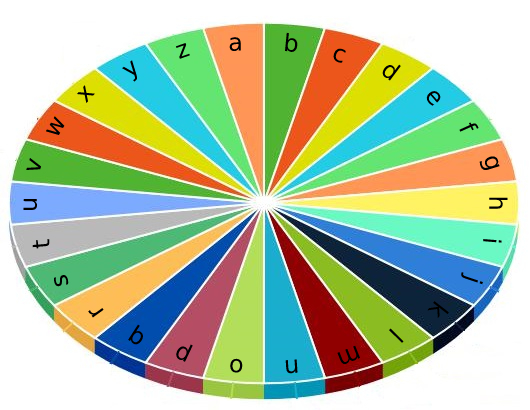Formatted question description: https://leetcode.ca/all/1974.html

# 1974. Minimum Time to Type Word Using Special Typewriter

Easy

## Description

There is a special typewriter with lowercase English letters 'a' to 'z' arranged in a circle with a pointer. A character can only be typed if the pointer is pointing to that character. The pointer is initially pointing to the character 'a'.Each second, you may perform one of the following operations:

• Move the pointer one character counterclockwise or clockwise.
• Type the character the pointer is currently on.

Given a string word, return the minimum number of seconds to type out the characters in word.

Example 1:

Input: word = “abc”

Output: 5

Explanation:

The characters are printed as follows:

• Type the character ‘a’ in 1 second since the pointer is initially on ‘a’.
• Move the pointer clockwise to ‘b’ in 1 second.
• Type the character ‘b’ in 1 second.
• Move the pointer clockwise to ‘c’ in 1 second.
• Type the character ‘c’ in 1 second.

Example 2:

Input: word = “bza”

Output: 7

Explanation:

The characters are printed as follows:

• Move the pointer clockwise to ‘b’ in 1 second.
• Type the character ‘b’ in 1 second.
• Move the pointer counterclockwise to ‘z’ in 2 seconds.
• Type the character ‘z’ in 1 second.
• Move the pointer clockwise to ‘a’ in 1 second.
• Type the character ‘a’ in 1 second.

Example 3:

Input: word = “zjpc”

Output: 34

Explanation:

The characters are printed as follows:

• Move the pointer counterclockwise to ‘z’ in 1 second.
• Type the character ‘z’ in 1 second.
• Move the pointer clockwise to ‘j’ in 10 seconds.
• Type the character ‘j’ in 1 second.
• Move the pointer clockwise to ‘p’ in 6 seconds.
• Type the character ‘p’ in 1 second.
• Move the pointer counterclockwise to ‘c’ in 13 seconds.
• Type the character ‘c’ in 1 second.

Constraints:

• 1 <= word.length <= 100
• word consists of lowercase English letters.

## Solution

The pointer is initially at 'a'. For each character, first move the pointer to the character, and then type the character. For moving the pointer to the character, calculate the absolute difference between the current character and the previous character, and determine whether to move counterclockwise or clockwise to make the time minimum. For typing the character, it always takes 1 second. Finally, return the minimum total time.

class Solution {
public int minTimeToType(String word) {
int time = 0;
char prev = 'a';
int length = word.length();
for (int i = 0; i < length; i++) {
char curr = word.charAt(i);
int difference = Math.abs(curr - prev);
difference = Math.min(difference, 26 - difference);
time += difference + 1;
prev = curr;
}
return time;
}
}